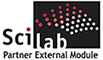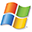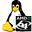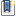ATOMS : Financial details

# Financial

Module is about risk measure and management, asset allocation, and pricingDetails
Version
1.0.1
Author
Francesco Menoncin
Owner Organization
Brescia University - Economics Department
Maintainers
Michael Baudin
Francesco Menoncin
Category
Dependency
Creation Date
September 28, 2012
Source created on
Scilab 5.4.x
Binaries available on
Scilab 5.4.x:Windows 64-bitWindows 32-bitLinux 64-bitLinux 32-bitMacOSX
Scilab 5.5.x:Windows 64-bitWindows 32-bitLinux 64-bitLinux 32-bitMacOSX
Install command
`--> atomsInstall("Financial")`
Report a bugDescription
```            The module is dedicated to finance. There are three main areas that are covered:
(i) risk measure and management, (ii) asset allocation, and (iii) pricing.

For what concerns the risk measure, some functions are dedicated to the
computation of Value at Risk (VaR) and Expected Shortfall (ES). Backtest is also
implemented in order to check the goodness of such risk measures. Both VaR and
ES are also computed in an Extreme Value Theory framework (EVT). Furthermore, it
is possible to estimate the parameters of the EVT density function (through
maximum likelihood). The Mean Excess Function for graphical study of an EVT
distribution is also implemented.
The interest rate risk is faced by functions aimed at computing duration,
convexity, and yield to maturity. Furthermore, Merton, Vasicek and Cox,
Ingersoll and Ross interest rate models are implemented together with the
estimation of their parameters. Parametric interpolation of the interest rate
curve is possible through both Svennson’s and Nelson-Siegel’s models.
Finally, some technical analysis indicators are implemented: Bollinger bands,
moving averages, Hurst index.

The asset allocation problem is faced by two functions which compute: (i) the
optimal portfolio minimizing the variance of its return and (ii) the optimal
portfolio minimizing the expected shortfall of its return. In both cases, the
portfolios with and without a riskless asset and with and without short selling
are computed.

Pricing problem is approached through functions aimed at: (i) computing the
spread on Interest Rate Swaps, (ii) computing the value of options in the Black
and Scholes framework (with Greeks and implied volatility), (iii) simulating
stochastic processes (through Euler discretization).

Features
--------

* backtest : Apply the backtest to Expected Shortfall, Value at Risk and a
Linear Spectral risk measure.
* bollinger : Plots the historical prices, the Bollinger bands, and the
b-percentage.
* bsgreeks : Compute the Greeks for Black and Scholes put and call options.
* bsimpvol : Compute the implied volatility in a Black and Scholes framework.
* bsoption : Compute the value of both a call and a put option in a Black and
Scholes framework.
* cfr : Compare and merge two or more time series according to dates.
* duration : Compute both duration and convexity of cash flows by using the
yield-to-maturity.
* esvarevt : Compute both Expected Shortfall and Value at Risk.
* esvarlin : Compute Expected Shortfall, Value at Risk and a Linear Spectral
risk measure on a set of assets.
* esvaroptim : Compute the optimal portfolio minimizing the Expected
Shortfall.
* euler : Simulate the solution of a system of stochastic differential
equation.
* evt : Estimate the parameters of the Generalized Pareto Distribution.
* gbm : Estimate the parameters of a Geometric Brownian Motion.
* hedge : Compute the hedge ratio between an asset and a derivative on that
asset.
* hurst : Compute the Hurst index on historical prices.
* interest : Estimate the parameters of three spot interest rate models (Merton
- Vasicek - CIR).
* irs : Compute both the spread and the value of the legs of a fix-for-floating
Interest Rate Swap.
* markowitz : Compute the optimal portfolio minimizing the variance.
* mef : Compute and draw the Mean Excess Function.
* movav : Compute and draw the moving average of a given time series.
* nelson_siegel : Estimate the parameters for the Nelson Siegel model of spot
interest rates.
* svennson : Estimate the parameters for the Svennson model of spot interest
rates.

```Files (3)Source code archive
```makes it available for Scilab 5.4
```OS-independent binary for Scilab 5.4.x
```Binary version
Automatically generated by the ATOMS compilation chain

```OS-independent binary for Scilab 5.5.x
```Binary version (all platforms)
Automatically generated by the ATOMS compilation chain

```News (0)Comments (0)Leave a comment
You must register and log in before leaving a comment.Email notifications
Send me email when this toolbox has changes, new files or a new release.